# Texas Go Math Grade 2 Lesson 1.6 Answer Key Word Form for Numbers

Refer to our Texas Go Math Grade 2 Answer Key Pdf to score good marks in the exams. Test yourself by practicing the problems from Texas Go Math Grade 2 Lesson 1.6 Answer Key Word Form for Numbers.

## Texas Go Math Grade 2 Lesson 1.6 Answer Key Word Form for Numbers

Explore

Write numbers to complete the chart. Then find and circle the word form of these numbers below.FOR THE TEACHER • Read the following problem. Chelsea finds this page in her book of math puzzles. How can Chelsea complete the page?Explanation: Word form is one way in which the concept of numbers can be written. Word form involves expressing numbers using words rather than numerals.

Math Talk
Mathematical Processes

Describe how to use words to write the number with, a 5 in the tens place and in the one’s place.
How to write numbers in word form:
Note: Learn to write numbers from 1 to 9. This is where it all starts. Learn these, and the rest gets much easier:
1. Understanding the place values: We can tell what each digit in a number means based on where it is.
2. Write the digits now, given 5 is in the tens place and the same number is in the one’s place.
3. Write that two digits. Look at the two digits of the number. This part is just like writing a normal two-digit number.
4. The number is 55. Write this as fifty-five.

Model and Draw

You can use words to write 3-digit numbers. First, look at the hundreds digit. Then, look at the tens digit and ones digit together.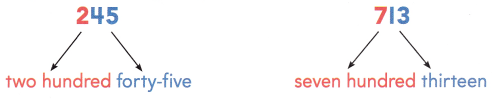Share and Show

Write the number using words.

Question 1.
506
Explanation:1. Understanding the place values: We can tell what each digit in a number means based on where it is.
2. Write the last two digits at the end of the number. This part is just like writing a normal two-digit number.
– 506: Start with just the 06 at the end. Write this as six because there is no number in the tens place so just write one’s place.
3. Write the hundreds place. The next digit is the hundreds place. This is an easy one. Just write the number in that place, then add the word “hundred.” Write this in front of your number.
– In 506, the 5 is in the hundreds place. Write this as five hundred.
4. Now we have five hundred six.

Question 2.
189
Explanation: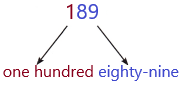1. Understanding the place values: We can tell what each digit in a number means based on where it is.
2. Write the last two digits at the end of the number. This part is just like writing a normal two-digit number.
– 189: Start with just the 89 at the end. Write this as eighty-nine
3. Write the hundreds place. The next digit is the hundreds place. This is an easy one. Just write the number in that place, then add the word “hundred.” Write this in front of your number.
– In 189, the 1 is in the hundreds place. Write this as one hundred.
4. Now we have one hundred eighty-nine.

Question 3.
328
Explanation:1. Understanding the place values: We can tell what each digit in a number means based on where it is.
2. Write the last two digits at the end of the number. This part is just like writing a normal two-digit number.
– 328: Start with just the 28 at the end. Write this as twenty-eight
3. Write the hundreds place. The next digit is the hundreds place. This is an easy one. Just write the number in that place, then add the word “hundred.” Write this in front of your number.
– In 328, the 3 is in the hundreds place. Write this as three hundred.
4. Now we have three hundred twenty-eight.

Write the number.

Question 4.
four hundred fifteen
Explanation:
1. Understanding the place values: We can tell what each digit in a number means based on where it is.
2. First write the hundreds place and tens place and then one’s place.
3. We read the numbers from left to write.
Question 5.
two hundred ninety-one
Explanation:
In the number 291, 2 is in the hundreds place, 9 is in the tens place, and 1 is in one’s place.
Question 6.
six hundred three
Explanation:
In the number 603, 6 is in the hundreds place, 0 is in the tens place, and 3 is in the one’s place.

Question 7.
eight hundred forty-seven
Explanation:
In the 847, 8 is in the hundreds place, 4 is in the tens place, and 7 is in the one’s place.

Problem Solving

Write the number.

Question 8.
six hundred forty-three
Explanation:
In the number 643, 6 is in the hundreds place, 4 is in the tens place, and 3 is in the one’s place.
– To convert words to numbers we have to know the place values and words of numbers.

Question 9.
nine hundred twelve
Explanation:
In the number 912, 9 is in the hundreds place, 1 is in the tens place, and 2 is in the one’s place.
– To convert words to numbers we have to know the place values and words of numbers.

Write the number using words.

Question 10.
632
Explanation:1. Understanding the place values: We can tell what each digit in a number means based on where it is.
2. Write the last two digits at the end of the number. This part is just like writing a normal two-digit number.
– 632: Start with just the 32 at the end. Write this as thirty-two
3. Write the hundreds place. The next digit is the hundreds place. This is an easy one. Just write the number in that place, then add the word “hundred.” Write this in front of your number.
– In 632, the 6 is in the hundreds place. Write this as six hundred.
4. Now we have six hundred thirty-two.

Question 11.
568
Explanation: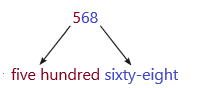1. Understanding the place values: We can tell what each digit in a number means based on where it is.
2. Write the last two digits at the end of the number. This part is just like writing a normal two-digit number.
– 568: Start with just the 68 at the end. Write this as sixty-eight
3. Write the hundreds place. The next digit is the hundreds place. This is an easy one. Just write the number in that place, then add the word “hundred.” Write this in front of your number.
– In 568, the 5 is in the hundreds place. Write this as five hundred.
4. Now we have five hundred sixty-eight.

Question 12.
321
Explanation: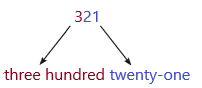1. Understanding the place values: We can tell what each digit in a number means based on where it is.
2. Write the last two digits at the end of the number. This part is just like writing a normal two-digit number.
– 321: Start with just the 21 at the end. Write this as twenty-one
3. Write the hundreds place. The next digit is the hundreds place. This is an easy one. Just write the number in that place, then add the word “hundred.” Write this in front of your number.
– In 321, the 3 is in the hundreds place. Write this as three hundred.
4. Now we have three hundred twenty-one.

Question 13.
H.O.T. Alma counts two hundred sixty-eight leaves. Which is another way to write this number? Circle your answer.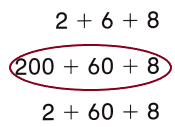Explanation:
The standard form of a number is 268
We can write in expanded form also:
The expanded form of a number 268 is 200+60+8
In mathematics, the expanded form of a number is defined as the notation. It is the way of writing the numbers to define the individual digit value. When the numbers are written using place value, it will create a new algebraic expression.
268:
2 is in the hundreds place.
6 is in the tens place.
8 is in the one’s place.

Question 14.
H.O.T. Multi-Step My 3-digit number has a 4 in the hundreds place. It has a greater digit in the tens place than in the ones place. The sum of the digits is 6.
What is my number?
Write the number using words.
Explanation:
We need to fill the three digits: –  –  –
Already given 4 is in the hundreds place:
4 – –
Now we need to fill the two blanks above. Here asked the sum of digits should be 6. This can be explained below:
*Since the first digit is 4, the sum of the last two digits must be 2. So they can either be two 1’s or a 0 and a 2.
In the question given that it has a greater digit in the tens place than in one’s place:
* The above rule out two 1’s. So that means 0 and 2 will occupy the next two places since the tens digit is greater it must be 2 and one’s digit is automatically 0. So the answer is 4 2 0.
Now if we add these three digits 4+2+0, we get 6 only.

Question 15.
Denzel has two hundred thirty-two crayons. Which number shows how many crayons Denzel has?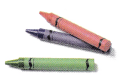(A) 223
(B) 322
(C) 232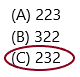Explanation:
Given in the word form: two hundred thirty-two
The number of crayons Denzel has=232
The word form two hundred thirty-two represents the number 232.
2 is in the hundreds place
3 is in the tens place
2 is in the one’s place.

Question 16.
Representations Together, Denzel and his brother have 53 crayons. Which is another way to write 5q3?
(A) three hundred ninety-five
(B) five hundred ninety-three
(C) nine hundred fifty-three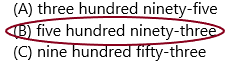Explanation:
1. Understanding the place values: We can tell what each digit in a number means based on where it is.
2. Write the last two digits at the end of the number. This part is just like writing a normal two-digit number.
– 593: Start with just the 93 at the end. Write this as ninety-three
3. Write the hundreds place. The next digit is the hundreds place. This is an easy one. Just write the number in that place, then add the word “hundred.” Write this in front of your number.
– In 593, the 5 is in the hundreds place. Write this as five hundred.
4. Now we have five hundred ninety-three.

Question 17.
Analyze Katie and her sister have six hundred twelve crayons. Which shows this number?
(A) 126
(B) 620
(C) 612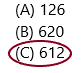Explanation:
Already given in the word form: Six hundred twelve.
The number of crayons Katie and her sister have=612
The word form six hundred twelve represents the number 612.
6 is in the hundreds place
1 is in the tens place
2 is in the one’s place.

Question 18.
TEXAS Test Prep There are five hundred thirty-seven chairs at the school. Which shows this number?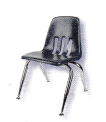(A) 507
(B) 537
(C) 357
Explanation:
Already given in the word form: five hundred thirty-seven
The number of chairs is there in school=537
The word form five hundred thirty-seven represents the number 537.
5 is in the hundreds place
3 is in the tens place
7 is in the one’s place.

TAKE HOME ACTIVITY • Ask your child to write the number 94o using words.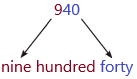First, learn 1 to 10 numbers, writing in words.
1. Understanding the place values: We can tell what each digit in a number means based on where it is.
2. Write the last two digits at the end of the number. This part is just like writing a normal two-digit number.
– 940: Start with just the 40 at the end. Write this as forty.
3. Write the hundreds place. The next digit is the hundreds place. This is an easy one. Just write the number in that place, then add the word “hundred.” Write this in front of your number.
– In 940, the 9 is in the hundreds place. Write this as nine hundred.
4. Now we have nine hundred forty.

### Texas Go Math Grade 2 Lesson 1.6 Homework and Practice Answer Key

Write the number using words.

Question 1.
292
Explanation:1. Understanding the place values: We can tell what each digit in a number means based on where it is.
2. Write the last two digits at the end of the number. This part is just like writing a normal two-digit number.
– 292: Start with just the 92 at the end. Write this as ninety-two.
3. Write the hundreds place. The next digit is the hundreds place. This is an easy one. Just write the number in that place, then add the word “hundred.” Write this in front of your number.
– In 292, the 2 is in the hundreds place. Write this as two hundred.
4. Now we have two hundred ninety-two.

Question 2.
467
Explanation: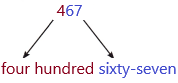1. Understanding the place values: We can tell what each digit in a number means based on where it is.
2. Write the last two digits at the end of the number. This part is just like writing a normal two-digit number.
– 467: Start with just the 67 at the end. Write this as sixty-seven.
3. Write the hundreds place. The next digit is the hundreds place. This is an easy one. Just write the number in that place, then add the word “hundred.” Write this in front of your number.
– In 467, the 4 is in the hundreds place. Write this as four hundred.
4. Now we have four hundred sixty-seven.

Question 3.
668
Explanation: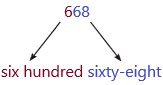1. Understanding the place values: We can tell what each digit in a number means based on where it is.
2. Write the last two digits at the end of the number. This part is just like writing a normal two-digit number.
– 668: Start with just the 68 at the end. Write this as sixty-eight.
3. Write the hundreds place. The next digit is the hundreds place. This is an easy one. Just write the number in that place, then add the word “hundred.” Write this in front of your number.
– In 668, the 6 is in the hundreds place. Write this as six hundred.
4. Now we have six hundred sixty-eight.

Write the number.

Question 4.
five hundred thirteen
Already word form is given. We need to convert the word form into a number form.
Five hundred thirteen.
We always read numbers from left to right.
The number form is 513.
5 is in the hundreds place
1 is in the tens place
3 is in the one’s place.

Question 5.
eight hundred twenty-four
Already word form is given. We need to convert the word form into a number form.
We always read numbers from left to right.
The number form is 824
8 is in the hundreds place
2 is in the tens place
4 is in the one’s place.

Question 6.
two hundred fifty-nine
Already word form is given. We need to convert the word form into a number form.
We always read numbers from left to right.
The number form is 259.
2 is in the hundreds place
5 is in the tens place
9 is in the one’s place.

Question 7.
three hundred forty-six
Already word form is given. We need to convert the word form into a number form.
We always read numbers from left to right.
The number form is 346.
3 is in the hundreds place
4 is in the tens place
6 is in the one’s place.

Problem Solving

Solve.

Question 8.
Adam counts one hundred ninety-six plants. Which is another way to write this number?
(A) 1 + 9 + 6
(B) 100 + 90 + 6
(C) 1 + 90 + 6Explanation:
The standard form of a number is 196
We can write in expanded form also:
The expanded form of a number 196 is 100+90+6
In mathematics, the expanded form of a number is defined as the notation. It is the way of writing the numbers to define the individual digit value. When the numbers are written using place value, it will create a new algebraic expression.
196:
1 is in the hundreds place.
9 is in the tens place.
6 is in the one’s place.

Lesson Check

Question 9.
Lindsey has one hundred twenty-three markers. Which number shows how many markers Lindsey has?
(A) 321
(B) 123
(C) 213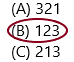Explanation:
The number of markers Lindsey has=123
Already given in the word form: one hundred twenty-three
The word form one hundred twenty-three represents the number 123.
1 is in the hundreds place
2 is in the tens place
3 is in the one’s place.

Question 10.
Together, Lindsey and her friend have 295 markers. Which is another way to write 295?
(A) five hundred ninety-two
(B) two hundred fifty-nine
(C) two hundred ninety-five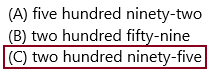Explanation: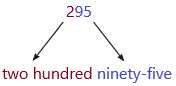1. Understanding the place values: We can tell what each digit in a number means based on where it is.
2. Write the last two digits at the end of the number. This part is just like writing a normal two-digit number.
– 295: Start with just the 95 at the end. Write this as ninety-five.
3. Write the hundreds place. The next digit is the hundreds place. This is an easy one. Just write the number in that place, then add the word “hundred.” Write this in front of your number.
– In 295, the 2 is in the hundreds place. Write this as two hundred.
4. Now we have two hundred ninety-five.

Question 11.
Tim and his brother collect bottle caps for a school project. So far, they have five hundred nineteen bottle caps. Which is another way to write the number?
(A) 591
(B) 159
(C) 519Explanation:
The number of markers Lindsey has=519
Already given in the word form: five hundred nineteen
The word form five hundred nineteen represents the number 519
5 is in the hundreds place
1 is in the tens place
9 is in the one’s place.

Question 12.
There are a great many desks at the school. The number has a 3 in the hundreds place. It has the same digit in the tens place. The sum of those two digits is in the ones place. How many desks are at the school?
(A) 336
(B) 363
(C) 360